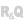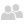• Overview
• Initializing a List Box control
• Initializing an element
• Populating a List Box control
• Selecting an element in a List Box control
• Selecting a row
• Setting the position on the last element
• Retrieving the element selected in a List Box control
• Retrieving the index of the selected element
• Getting the value of the selected element
• Iterating over the elements of a List Box control
• Properties specific to List Box controls
WINDEVWEBDEVWINDEV MobileOthersHandling List Box controls programmatically (prefix syntax)
Overview
WINDEV, WEBDEV and WINDEV Mobile allow you to programmatically handle List Box controls. To do so, use the variable of the List Box control in the code. This variable is of type numeric.
The variable of the List Box control:
• corresponds to the name of the List Box control.
• is initialized with the index of the element selected in the control.
To handle an element of the List Box control, use the following notation:
<List Box control> [<Element index>]
Remark: There are multiple WLanguage functions specific to List Box controls. For more details, see functions for handling List Box controls.
Initializing a List Box control

Initializing an element

To initialize an element in a List Box control, use <List Box>.Add.
Remark: To modify an element in a List Box control, use the following syntax:
<List Box control>[Index] = <Value>
For example:
// Modify elements
LIST_LIST1 = "MOORE"

Populating a List Box control

To initialize a List Box control, simply use an iteration loop to initialize all the elements in the control.
Index is int
FOR Index = <Start position> TO <End position>
END
Example:
i is int
FOR i = 1 TO 52
END
Selecting an element in a List Box control

Selecting a row

To select the element at row <Index>, use:
• the direct assignment:
<List Box control> = Index
• the <List Box>.SelectPlus function:
<List Box control>.SelectPlus(<Index>)

Setting the position on the last element

To set the position on the last element of the List Box control, use:
Retrieving the element selected in a List Box control

Retrieving the index of the selected element

To retrieve the index of the selected element, use one of the following syntaxes:
Index = <List Box control>
• the <List Box>.Select function:
Index = <List Box control>.Select()
Remark: For multi-selection List Box controls, <List Box>.Select is used to retrieve the selected elements.
// Retrieve the elements of a multi-selection List Box control
Rank is int = 0  // selection number
Index is int = 0  // index of the selected element
LOOP
Rank = Rank + 1
Index = LIST_LIST1.Select(Rank)
IF Index = - 1 THEN BREAK
END

Getting the value of the selected element

To retrieve the value of selected element, use one of the following syntaxes:
• Syntax 1:
Index is int = <List Box control>
<Variable> = <List Box control>[Index]
• Syntax 2:
<Variable> = <List Box control>[<List Box control>]
• Syntax 3: DisplayedValue property (in server code only)
Variable> = <List Box control>[Index].DisplayedValue
Caution: The stored value can be different from the displayed value. To get the stored value corresponding to one of the rows in the List Box control, use the StoredValue property.
Iterating over the elements of a List Box control
The FOR EACH statement performs different types of iterations on List Box controls:
• Iterating over the elements in the control. The following syntax is used:
FOR EACH ROW [<Index> [, <Counter>]] OF <List Box control>
...
END
where:
• <Index>: Optional Integer variable. For each iteration, contains the index of the current row.
• <Counter>: Optional Integer variable. This variable counts the number of iterations.
• Iterate over the selected elements. The following syntax is used:
FOR EACH SELECTED ROW [<Index>, [<Counter>]] OF <List Box control>
...
END
where:
• <Index>: Integer variable. For each iteration, contains the index of the current selected row.
• <Counter>: Optional Integer variable. This variable counts the number of iterations (number of selected rows for example).
Properties specific to List Box controls
The following properties are specific to List Box controls:
 All types of List Box controls HorizontalAlignment Gets and sets the horizontal alignment of the elements in the List Box control. LineHeight Gets and sets the height of the rows in a List Box control. Memory Indicates if the specified List Box control is populated programmatically or is based on a data file. Count Gets the number of rows in a List Box control. FillType Indicates how a control is populated (programmatically, from a data file or from a variable). Applies to Table, List Box, ListView, Combo Box and Looper controls. DisplayedValue Gets the value of the current row or the value of a specific row in the List Box control. StoredValue Gets the value currently stored when selecting a row in the List Box control. Empty Indicates if a List Box control is empty. List Box control populated programmatically only InitialContent Gets the initial content of a List Box control populated programmatically. Sorted Indicates if a List Box control populated programmatically is sorted, or sorts the control. List Box control based on a data file only BrowsedFile Gets or sets the data file or query used to display records in List Box controls. AutoBrowse Indicates if a List Box control is looped through automatically or programmatically. DisplayedItem Gets and sets the item displayed in a List Box control. StoredItem Gets and sets the stored item of a List Box control. BrowsedItem Gets and sets the item used to automatically loop through List Box controls.

For a complete list of WLanguage properties that can be used with a List Box control, see Properties associated with List Box controls.
Minimum version required
• Version 23